### IMO Shortlist 1978 problem 17

Kvaliteta:
Avg: 0,0
Težina:
Avg: 0,0
Prove that for any positive integers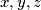$x, y, z$ with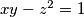$xy-z^2 = 1$ one can find non-negative integers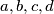$a, b, c, d$ such that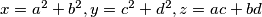$x = a^2 + b^2, y = c^2 + d^2, z = ac + bd$.
Set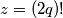$z = (2q)!$ to deduce that for any prime number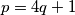$p = 4q + 1$,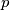$p$ can be represented as the sum of squares of two integers.
Izvor: Međunarodna matematička olimpijada, shortlist 1978